# KSEEB Solutions for Class 9 Science Chapter 12 Sound

## Karnataka Board Class 9 Science Chapter 12 Sound

### KSEEB Solutions Class 9 Science Chapter 12 Intext Questions

Question 1.
How does the sound produced by a vibrating object in a medium reach your ear?
Sound is produced by vibrating objects. When an object vibrates, it sets the particles of the medium around it vibrating. The particles do not travel all the way from the vibrating object in the ear. A particle of the medium in contact with the vibrating objects is first displaced from its equilibrium position.

It then exerts a force on the adjacent particle. As a result of which the adjacent particle. As a result of which the adjacent particle get displaced from its position at rest. After displacing the particle comes back to its original position. This process continues in the medium in the sound reaches our ears.

Question 2.
Explain how sound is produced by your school bell?
The bell produces the sound when it is struck by air hammer when the bell is struck by a hammer it starts vibrating. Since the vibrating objects produce sound. So the bell produces sound.

Question 3.
Why are sound waves called mechanical waves?
Sound waves are called mechanical waves because they are produced by the motion of particle of a medium. Hence sound waves are called mechanical waves.

Question 4.
Suppose you and your Mend are on the moon. Will you be able to hear any sound produced by your Mend?
I will not be able to hear any sound produced by my friend because the sound waves require some material medium like air to travel. There is no atmosphere or air on the moon. So the sound produced by my friend will not reach me and I will not be able to hear.

Question 5.
Which wave property determines
(a) loudness
(b) pitch?
(a) The loudness or softness of a sound is determined by its amplitude.
(b) Pitch of the sound is determined by its frequency.

Question 6.
Guess which sound has a higher pitch: guitar or car horn?
Guitar has a higher pitch

Question 7.
What are the wavelength, frequency, time period, and amplitude of a sound wave?

1. Wavelength: The distance travelled by the wave during one complete oscillation is called wavelength of the wave.
2. Frequency: The number of oscillations made by the wave in one second is called the frequency of the wave.
3. Time period: The time taken by the wave to complete one oscillation is called the amplitude of the wave.
4. amplitude: The maximum displacement of the wave from its mean or equilibrium position is called the amplitude of the wave.

Question 8.
How are the wavelength and frequency of a sound wave related to its speed?
The relation between wavelength (λ), frequency (ν) and speed of the wave (v) is
υ = λv.

Question 9.
Calculate the wavelength of a sound wave whose frequency is 220 Hz and speed is 440ms-1 in a given medium.
Frequency v = 220 Hz,
Speed u = 440 ms-1 Wavelength, l = ?
From the relation υ = vλ
440 = 220A.
∴ λ = $$\frac{440}{220}$$ = 2.0 m

Question 10.
A person is listening to a tone of 500 Hz sitting at a distance of 450 m from the source of the sound. What is the time interval between successive compressions from the source?
The time interval between two successive compressions or rarefactions is equal to the time period of the wave.
∴ Required time interval = time period
= $$\frac{1}{\text { Frequency }}=\frac{1}{500}$$ = 0.002 S
= 2 × 10-3 S = 2ms.

Question 11.
Distinguish between loudness and intensity of sound.

 Loudness Intensity Loudness is a measure of the response of the ear to the sound. The amount of sound energy passing each second through unit area is called the intensity of sound.

Question 12.
In which of the three media, air, water or iron, does sound travel the fastest at a particular temperature?
In these three media, sound travel in iron fastest at a particular temperature.

Question 13.
An echo returned in 3 S. What is the distance of the reflecting surface from the source, given that the speed of sound is 342ms?
Echo returns in 3S. Therefore time taken by sound to travel from the source to the reflecting surface
t = $$\frac{3}{2}=$$ = 1.5 S
Speed v = 342ms-1
∴ Distance of reflecting surface from the source = Speed × Time
= 342 × 1.5 = 513 m

Question 14.
Why are the ceilings of concert halls curved?
Generally, the ceilings of concert halls, are curved so that sound after reflection reaches all corners of the hall.

Question 15.
What is the audible range of the average human ear?
The audible range of sound for human beings extends from about 20Hz to 20000Hz.

Question 16.
What is the range of frequencies associated with
(a) Infrasound
(b) Ultrasound
(a) Infrasound: Sounds of frequencies below 20 Hz are called infrasonic sound or infrasound.
(b) Ultrasound: Frequencies higher than 20KHz are called ultrasound or ultrasonic sound.

Question 17.
A submarine emits a sonar pulse, which returns from an underwater cliff in 1.02s. If the speed of sound in saltwater is 1531 m/s how far away is the cliff?
Speed of sound in seawater, v = 1531 m/s.
Distance travelled ultrasonic sound = 2 × depth sea = 2d.
Here d means depth of sea
2d = Speed of sound × time
= 1531 m/s × 1.02
= 1561.62 m
d = 1561.62/2 = 780.81 m.
Cliff is 780.81 m away from the sea.

### KSEEB Solutions for Class 9 Science Chapter 12 Textbook Exercises

Question 1.
What is sound and how is it produced?
Sound is a form of energy – the sound is produced due to the vibration of different objects.

Question 2.
Describe with the help of a diagram, how compressions and rarefactions are produced in air near a source of the sound.
Air is the most common medium through which sound travels. When a vibrating object moves forward, it pushes and compresses the air in front of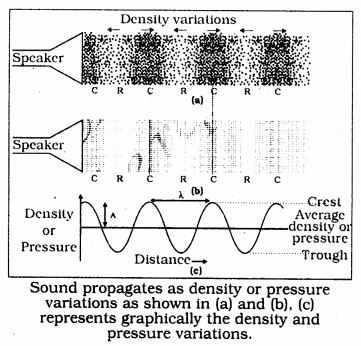it creating a region of high pressure. This region is called a compression (c) as shown in Fig. This compression starts to move away from the vibrating object when the vibrating object moves backward, it creates a region of low pressure called rarefaction (R) as shown in Fig. As the object moves back and forth rapidly, a series of compressions and rarefactions is created in the air.

Question 3.
Cite an experiment to show that sound needs a material medium for its propagation.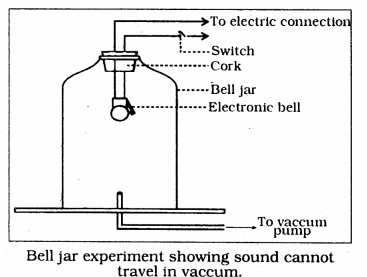Experiment: Take an electric bell and an airtight glass bell jar. The electric bell is suspended inside the airtight bell jar. The bell jar is connected to a vacuum pump as shown in figure. If we press the switch we will be able to hear the bell. Now start the vacuum pump. When the air in the jar is pumped out gradually, the sound becomes painter, although the same current is passing through the bell. After sometime when less air is left inside the bell jar we will near a feeble sound. If the air is removed completely we can’t hear the bell. From this experiment, we conclude that sound needs a material medium for its propagation.

Question 4.
Why is a sound wave called a longitudinal wave?
Sound waves are called longitudinal waves because the particles do not move from one place to another but they simply oscillate back and forth about their position of rest.

Question 5.
Which characteristic of the sound helps you to identify your Mend by his voice while sitting with others in a dark room?
The quality or timbre of a sound is the characteristic of the sound which helps to us to identify our friend by his voice while sitting with others in a dark room.

Question 6.
Plash and thunder are produced simultaneously. But thunder is heard a few seconds after the flash is seen. Why?
Thunder is heard a few seconds later after the flash because the speed of light in the atmosphere for air is 3 × 108 ms-2 which is very high, as compared to the speed of sound which is only 330 ms-1. So the sound of thunder reaches us later than the flash.

Question 7.
A person has a hearing range from 20Hz to 20KHz. What are the typical wavelengths of sound waves in air corresponding to these two frequencies? Take the speed of sound in air as 344 ms-2).
The relation between speed (v), wavelength (X) and frequency (v) of a wave, we have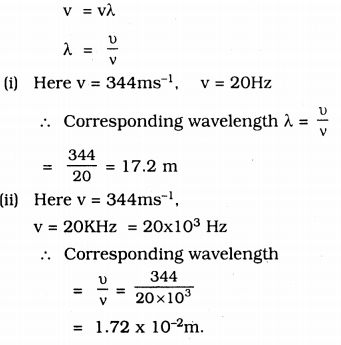Question 8.
Two children are at opposite ends of an alluminium rod. One strikes the end of the rod with a stone. Find the ratio or times taken by the sound wave in air and in alluminium to reach the second child?
Let l = length of the rod.
Time taken by the sound to travel through the alluminium rod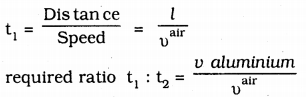Question 9.
The frequency of a source of sound is 100Hz. How many times does it vibrate in a minute?
Frequency = 100Hz.
From the definition of frequency, we can say that Number of oscillations in 1 min or 60 Sec. = 100 × 60
= 6000 = 6 × 103.

Question 10.
Does sound follow the same laws of reflection as light does? Explain.
Yes. Sound waves follow the same laws of reflection as light does. The directions in which the sound is incident and is reflected make equal angles with the normal to the reflecting surface at the point of incidence and three are in the same plane.

Question 11.
When a sound is reflected from a distant object, an echo is produced, Let the distance between the reflecting surface and the source of sound production remains the same. Do you hear echo sound on a hotter day?
The time taken by echo to be heard
t = $$\frac{2 \mathrm{d}}{v}$$
where d = distance between the reflecting surface and source of the sound and v = speed of sound in air. As we know the speed of sound increases with an increase in temperature. So on a hotter day speed of sound will be higher, so the time after which echo is heard will decrease. If the time taken by the reflected sound is less than 0.15 after the production of the original sound, then echo is not heard.

Question 12.
Give two practical applications of reflection of sound waves.
(1) Megaphone (2) Hearing aid are two practical applications of reflection of sound waves.

Question 13.
A stone is dropped from the top of a tower 500 m high into a pond of water at the base of the tower. When is the splash heard at the top? Given, g= 10ms-2 and speed of sound = 340ms-1)
Time after which splash is heard = Time taken by the stone to reach the pond + Time taken by the splash sound to reach the top of tower.
(i) For the time taken by the stone to reach the pond.
Here u = 0 (∵ stone is dropped from rest)
From equation of motion against gravity.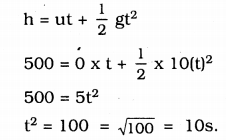(ii) Time taken by splash sound to reach the top of lower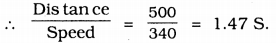∴ Time after which splash is heard = 10 + 1.47 = 11.47 S.

Question 14.
A sound wave travels at a speed of 339ms-1. If its wavelength is 1.5 cm. What is the frequency of the wave? Will it be audible?
Speed = 339 ms-1
Wavelength λ = 1.5 cm. = 1.5 × 102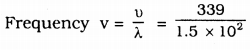= 22600 Hz.
This frequency is greater than 20000 Hz. So it will not be audible. For an audible range of the human ear is 20Hz to 20000 Hz.

Question 15.
What is reverberation? How can it be reduced?
The phenomenon of propagation of original sound due to the multiple reflections of sound waves even after the source of sound and stops producing sound is called reverberation. It can be reduced by covering the roofs and walls of the hall by absorbing materials.

Question 16.
What is loudness of sound? What factors does it depend on?
Loudness of a sound is a subjective quantity. It causes an unpleasant effect on air. Loudness depends on

1. the amplitude of the vibrating body and
2. The sensitivity of the human ear.

Question 17.
Explain how bats use ultrasound to catch a prey.
Bats emit ultrasonic waves and search their food. Ultrasonic waves having higher pitch reflects from prey and reaches ears of the Bat. Nature of reflection indicates to Bat regarding position and type of prey.

Question 18.
How is ultrasound used for cleaning?
Objects to be cleaned are placed in a cleaning solution and ultrasonic waves are sent into the solution. Due to high frequency, the particles of dust, grease, and dirt get detached and drop out. The objects thus get thoroughly cleaned.

Question 19.
Explain the working and application of a sonar.
Working: Sonar consists of a transmitter and a detector and is installed in a boat or a ship as shown in Fig. The transmitter produces and transmits ultrasonic waves. These waves travel through water and after striking the object on the seabed, get reflected back and are sensed by the detector.

The detector converts the ultrasonic waves into electrical signals which are appropriately interpreted. The distance of the object that reflected the soundwave can be calculated by knowing the speed of sound in water and the time interval between the transmission and reception of the ultrasound. Let the time interval between transmission and reception of ultrasound signal be t and the speed of sound through seawater be v. The total distance, 2d travelled by the ultrasound is then, 2d = vxt.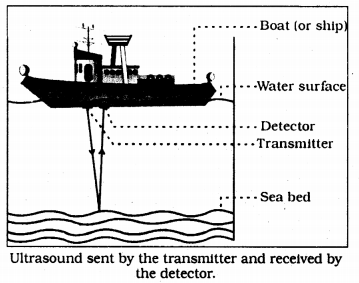Applications: The sonar technique is used to determine the depth of the sea and to locate underwater hills, valleys, submarine, icebergs, sunken ship etc.

Question 20.
A sonar device on a submarine sends out a signal and receives an echo 5s later. Calculate the speed of sound in water if the distance of the object from the submarine is 3625 m.
Time taken by submarine to sends out a signal and receives an echo,
t = $$\frac{5}{2}$$ = 2.5s.
distance of the object from the submarine = 3625m.
∴ Speed of sound in water,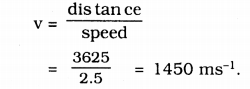Question 21.
Explain how defects in a metal block can be detected using ultrasound.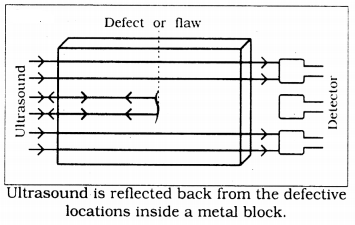The cracks or holes inside the metal blocks, which are invisible from outside reduces the strength of the structure Ultrasonic waves are allowed to pass through the metal block and detectors are used to detect the transmitted waves. If there is even a small defect, the ultrasound gets reflected back indicating the presence of the flaw or defect.

Question 22.
Explain how the human ear works.
Working on the human ear. The outer ear is called ‘pinna’. It collects the sound from the surroundings. The collected sound passes through the auditory canal. A the end of the auditory canal there is a thin membrane called the eardrum or tympanic membrane. When compression of the medium reaches the eardrum the pressure on the outside of the membrane increases and forces the eardrum inward.

Similarly, the eardrum moves outward when a rarefaction reaches it. In this way, the eardrum vibrates. The vibrations are amplified several times by three bones (the hammer, anvil, and stirrup) in the middle ear. The middle ear transmits the amplified pressure variations received from the sound wave to the inner ear. In the inner ear, the pressure variations are turned into electrical signals by the cochlea. These electrical signals are sent to the brain via the auditory nerve and the brain interprets them as sound.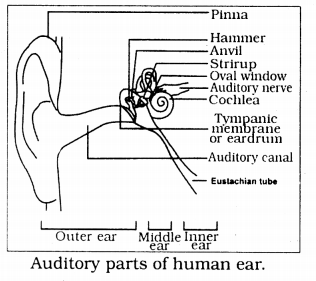### KSEEB Solutions for Class 9 Science Chapter 12 Additional Questions and Answer

Question 1.
What is a wave?
A wave is a disturbance that moves through a medium -when the particles of the medium set neighbouring particles into motion.

Question 2.
What type are sound waves?
Sound waves are mechanical waves (longitudinal waves).

Question 3.
What is S.I. unit of a sound wave?
Hertz (Hz).

Question 4.
What do you mean by tone?
A sound of a single frequency is called a tone.

Question 5.
What is the intensity of sound?